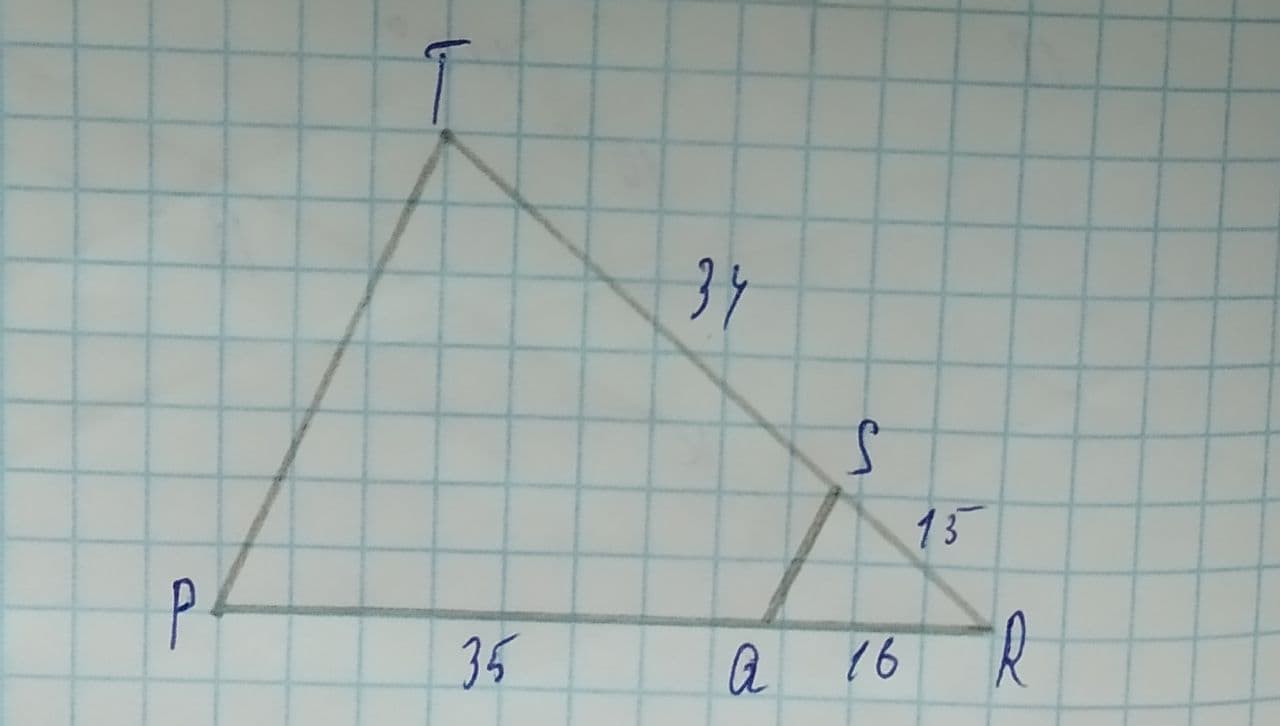# Given the diagram, determine whether line OS is parallel to line PT.Cem Hayes 2021-08-11 Answered
Given the diagram, determine whether line OS is parallel to line PT.1.yes, parallel
2.unable to determine
3.no, not parallel

• Questions are typically answered in as fast as 30 minutes

### Plainmath recommends

• Get a detailed answer even on the hardest topics.
• Ask an expert for a step-by-step guidance to learn to do it yourself.unett

Solution:
TP would be parallel to SQ if and only if the angle $$\displaystyle\angle{T}{P}{R}\stackrel{\sim}{=}\angle{S}{Q}{R}$$
To prove the, we shall use the similarity between the triangle TPR and SQR.
Using the property of similarity, if the the two triangles are similar, then,
$$\displaystyle{\frac{{{T}{S}}}{{{S}{R}}}}={\frac{{{P}{Q}}}{{{Q}{R}}}}$$
Now we shall check this by putting the values given, we get,
$$\displaystyle{\frac{{{34}}}{{{15}}}}\ne{\frac{{{35}}}{{{16}}}}$$
Hence the line are not parallel.